Next: 7. Photoresist Bake and Up: 6.5 Discussion Previous: 6.5.2 Limitations

## 6.5.3 Comparison with the Waveguide Method

Since the waveguide model is the second frequency domain method close similarities exist to the differential method. In fact we will show that the waveguide model equals the differential method if a zeroth-order vertical discretization scheme is used for the solution of the BVP. Hence the differential method comprises the waveguide model and is thus superior to it.

For that we reconsider (6.32). By comprising the four lateral vectors ex(z), ey(z), hx(z), and hy(z) carrying the relevant Fourier coefficients (cf. (6.30)) into two new vectors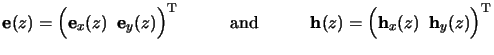(6.59)

we can rewrite the ODE system (6.32) to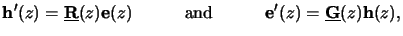(6.60)

whereby the two matrices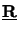(z) and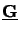(z) are given by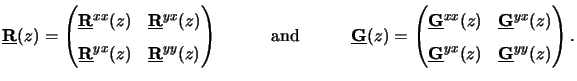(6.61)

In the waveguide method the medium is divided into thin layers with vertically constant refractive index [8,190,69,73,186,187,188]. The two matrices are assumed to be constant within such a layer, i.e., for z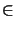[zl, zl + 1]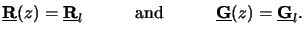(6.62)

One vector of unknowns, e.g., h(z), can thus simply be eliminated from the ODE system (6.89). By differentiating the left equation of (6.89) and inserting the right equation of (6.89) we obtaing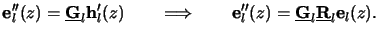(6.63)

The system matrix of the reduced second-order ODE system has a constant coefficient matrix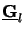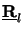. We set it equal to the square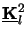of a matrix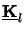, i.e., the NODE/2-dimensional second-order ODE system(6.64)

has to be solved within one layer z[zl, zl + 1]. This ODE system corresponds to the Helmholtz equation (4.2), whereby the wavenumber k is replaced by the matrix. Note that the system matrix has to be normal to write it as the squareof another matrix since only then an eigenvalue decomposition exists, and a matrixcan be found so that=.h The existence proof of the normal property ofas well as the calculation of the square root=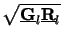can both be performed with the help of (6.22). Since we are only interested in a theoretical comparison the actual numerical evaluation is of minor interest. The formal solution of (6.93) is given by [203, pp. 937-947]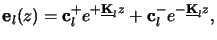(6.65)

whereby the matrix exponential exp(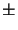z) can be calculated with the methods described in [202, pp. 572-577].

The solution (6.94) of the ODE system (6.93) corresponds to the solution of the eigenvalue problem occurring in the waveguide model [187, eq. (33)]. Since the matrix exponential can also be evaluated by an eigenvalue decomposition,i both methods--waveguide and zeroth-order differential--involve the same numerical operations and thus require the same computational costs. All other steps like matching the solutions of adjacent layers to determine the coefficient vectors cl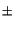of (6.94) and thereby propagating the solution through the whole simulation interval are equal. This proves theoretically that the differential method comprises the waveguide model as a sub-class, i.e., as the zeroth-order approximation.

As a direction for future work it would be interesting to compare both methods numerically. For a fair and correct benchmark a zeroth-order discretization scheme should be implemented for the differential method. This can be done most efficiently by determining the solution (6.94) of (6.93) since otherwise the higher order discretization is compared with the zeroth-order discretization of the waveguide model. Our implementation is not optimized for that purpose and the benchmark could potentially yield misleading results.

#### Footnotes

... obtaing
Also for vertically dependent matrices the vector h(z) can be eliminated from (6.89) yielding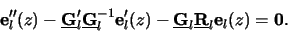Future research has to be done to investigate whether a direct solution of the above second-order ODE is advantageous to our implementation which solves the corresponding first-order ODE.
....h
The eigenvalue decomposition of a normal matrix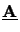is given by=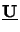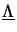, wherebyis a unitary matrix comprising the eigenvectorsuj =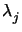uj andis a diagonal matrix with the eigenvaluesas diagonal entries. The square root is defined as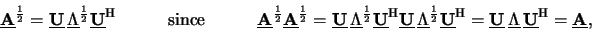whereby the unitary property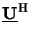=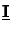ofhas been used and the square root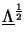of the diagonal matrixis simply given by taking the square root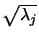of the diagonal entries. Numerically more efficient and stable methods exist to calculate the square root [202, pp. 572-577]. Note that any matrix polynomial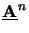can be calculated similarly to the square root, and thus a broad class of functions--including transcendental ones like the exponential--can be calculated from their Taylor series expansions.
... decomposition,i
See footnote  f on previous page.Next: 7. Photoresist Bake and Up: 6.5 Discussion Previous: 6.5.2 Limitations
Heinrich Kirchauer, Institute for Microelectronics, TU Vienna
1998-04-17